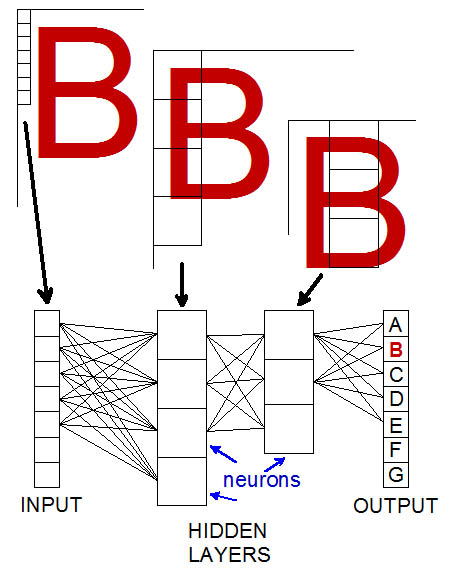# Definition: convolutional neural network

A widely used neural network that recognizes patterns. The convolutional neural network (CNN) breaks the input image into pixels and connects them to a series of neuron layers, each of which sees the image in a different location. The output layer is the best interpretation of the input. See neural network and AI.Character Recognition
In this simplistic example, the input images are random characters and the output is the letter of the alphabet. Each input pixel (greatly exaggerated in this example) is connected to every neuron in the next layer and so on all the way to the output layer. The interconnections are mathematically weighted and computed in each layer.There Are Many Kinds of CNNs
Neural networks can be complex. These are examples of convolutional architectures from the Asimov Institute. (Images courtesy of Fjodor van Veen and Stefan Leijnen (2019). The Neural Network Zoo, www.asimovinstitute.org/neural-network-zoo)